amortization.com Ltd.
Burlington, Ontario

905-639-0374
905-407-7988

info@amortization.comLess than Prime Percent

A lender offers a mortgage two percentage points below Prime for the first six months, and regular 6 year term rates for the remaining five and half years, providing you keep your mortgage with them for the 6 years (sign a 6 year term). Some lenders will offer less off the PRIME rate and a longer introduction period. For example, one percentage point below Prime but for a one and a half year introduction period. These lending ploys are smoke and mirrors. Most people do not have the time, software or mathematical knowledge required to evaluate these options. The lenders offering these smoke and mirror options are banking on the borrowers ignorance of mathematics (no pun intended). This type of confusion could easily be eliminated if all lenders provided effective interest rates (EIR) for all financial transactions. EIRs provide a simple means of comparing apples and oranges.

QUESTION 1: Do you know the overall interest rate if you accepted such an offer? If you knew the overall interest rate, because of the less than Prime introduction period, you could shop around and compare.

QUESTION 2: How much will you actually save during the 6 years if you accept such an offer? There are two reasons for evaluating the mortgage only to 6 years. Firstly, the term is over in 6 years and you do not know what interest rates will be in 6 years. Second, most North Americans sell their home every six years on average.

EXAMPLE: Using a \$100,000 mortgage that would normally be offered at an annual interest rate of 7% and amortized for 30 years. Monthly compounding is used thus the example is applicable to both Canadian and American borrowers. The initial data below is filled in as if the entire mortgage was to be at 7%.

(Screenshot 1)

DIGRESSION (Screenshot 2)

The initial Annual Interest Rate, typed into the calculator box, of 12% (1% per month) calculates the \$100 interest portion of Pmt #1. The 6% annual interest rate, shown in green (.5% per month) typed into the Int% cell of pmt #2 calculates the \$40 interest portion of pmt #3 (.005 x \$8000 = \$40). The 6% annual interest rate, shown in orange (.5% per month) typed into the Int% cell of pmt #5 calculates the \$25 interest portion of pmt #6 (.005 x \$5000 = \$25). The prepayment figures in yellow where specifically chosen so the interest calculations were easy to see. This digression will help one understand why an extra payment was inserted at the start of the amortization schedule example shown below.

Because the interest calculation for the very first payment in the amortization schedule is based upon 7%, the initial data, we can employ a unique feature in the MORTGAGE2 PRO PRO program. Place the cursor on the very first payment line of the schedule and insert a payment by clicking on the yellow pencil symbol. The new schedule will appear as below, (inserted payment shown in light blue);

(Screenshot 3)

Change the interest rate to 5% for the first six months as shown below.

Now make all the payments zero in the amortization spreadsheet, down to and including payment 73. This is actually a unique feature of MORTGAGE2 PRO PRO as it is generating a negative amortization schedule which has the same effect as doing a future value calculation on the monthly cash flow.

The value (in green) \$150,505.51 is the future value of a series of payments of 665.30 appreciating at 5% for the first six months and appreciating at 7% for the last 66 months.

Using any financial calculator it is now possible to calculate the overall interest rate over the 72 month period, by doing a variation on the Present Value (PV) / Future Value (FV) keys.

set PV= 100,000 FV= 150,505.51 n=6 years Calculate i%=7.05132

The interest calculated, 7.05132 (i%) represents the effective interest rate (EIR). The EIR is always greater than the stated annual interest rate (AIR) due to the method of compounding selected. By trial and error and typing in various Annual Interest Rates (AIR), starting with 6.7% on the MORTGAGE2 PRO calculator it does not take long to see that an AIR of 6.8332% with monthly compounding is exactly the same as an EIR of 7.05132%.

ANSWER to QUESTION 1 If the ABC Lending Co. offered you a different annual interest rate of 6.8332% using monthly compounding it would be exactly the same as this 5% for the first six months smoke and mirrors option. To be consistent, in vernacular, you could also call this stated annual interest rate of 6.8332% with monthly compounding, the overall interest rate as mentioned early in the example. In a perfectly simple world all you would need to know, in order to compare this smoke and mirror option, is the EIR of 7.05132% vs 7.2290%. When you borrow any money you are always paying the EIR because that is the rate you would be getting if you borrowed money from yourself and reinvested each monthly payment at the same rate payable to yourself (this is called deemed reinvestment).

The 7.2290 is the EIR of the mortgage at 7% with monthly compounding, over the 72 months.

Further proof of the relationship between compounding and effective interest rates.

You can easily see below that \$655.30 per month reinvested at 6.8332% with monthly compounding accumulates to \$150,505 after 72 months.

You can also easily see below that \$655.30 per month reinvested at 7.05132% with annual compounding accumulates to \$150,505 after 72 months. The two plans are mathematically identical (it is very difficult to get the accumulations to match to the exact penny due to slight rounding differences).

ANSWER to QUESTION 2 Your savings would be \$1,480.29 at the end of the 72 months.

This was the easy question. Simply compare the balance owing after 72 monthly payment of \$665.30 before (\$92,691.77) and after (\$91,211.48) the 5%/6 month deal. The is valid because the two scenarios have exactly 72 monthly payments each at \$665.30 per month.

BEFORE

AFTER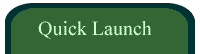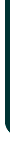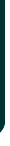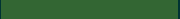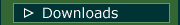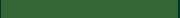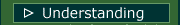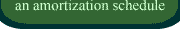VIDEOSamortizationdotcom Mortgage Calculator for iPhone

Introduction to Canadian and American Mortgages

Seminar on prepaying principal (Part A)

Seminar on prepaying principal (Part B)

Global TV Interview regarding 40 Year MortgagesLook for this logo on the Apple Store!

 < Go Back